# Linear Algebra and Matrices Linear Algebra and Matrices

• Slides: 10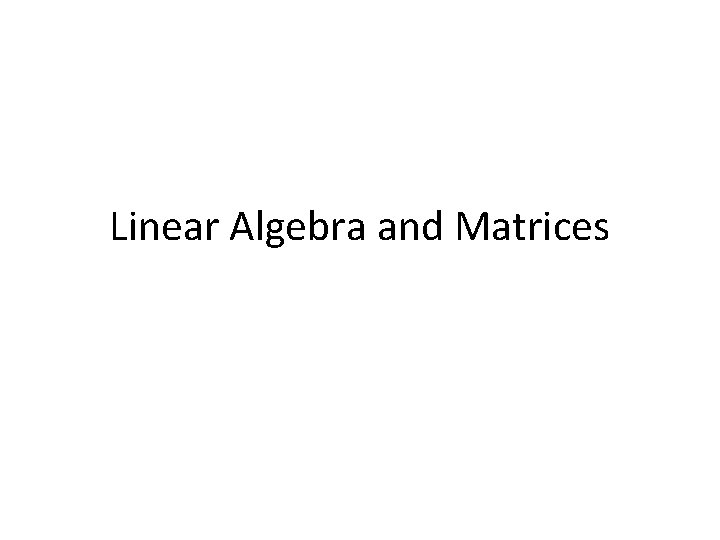Linear Algebra and Matrices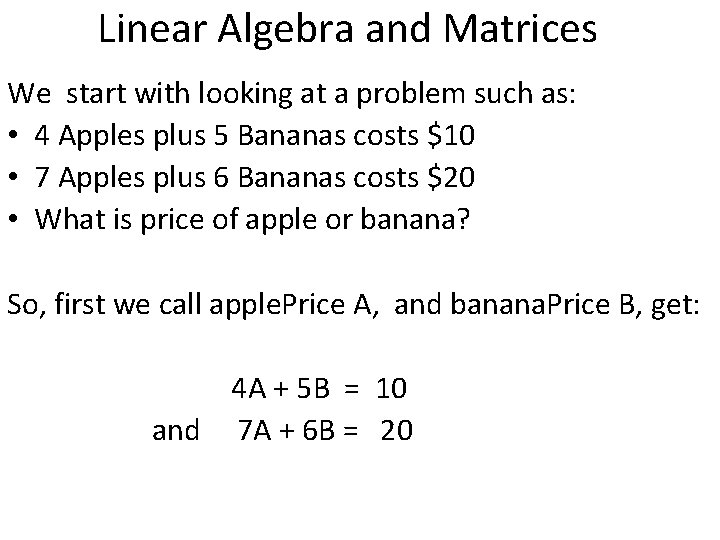Linear Algebra and Matrices We start with looking at a problem such as: • 4 Apples plus 5 Bananas costs \$10 • 7 Apples plus 6 Bananas costs \$20 • What is price of apple or banana? So, first we call apple. Price A, and banana. Price B, get: 4 A + 5 B = 10 and 7 A + 6 B = 20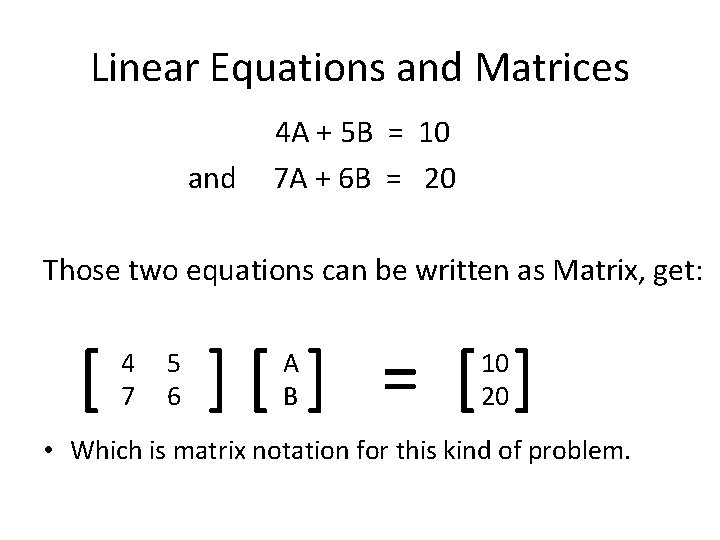Linear Equations and Matrices and 4 A + 5 B = 10 7 A + 6 B = 20 Those two equations can be written as Matrix, get: [ 4 7 5 6 ][ ] = [ ] A B 10 20 • Which is matrix notation for this kind of problem.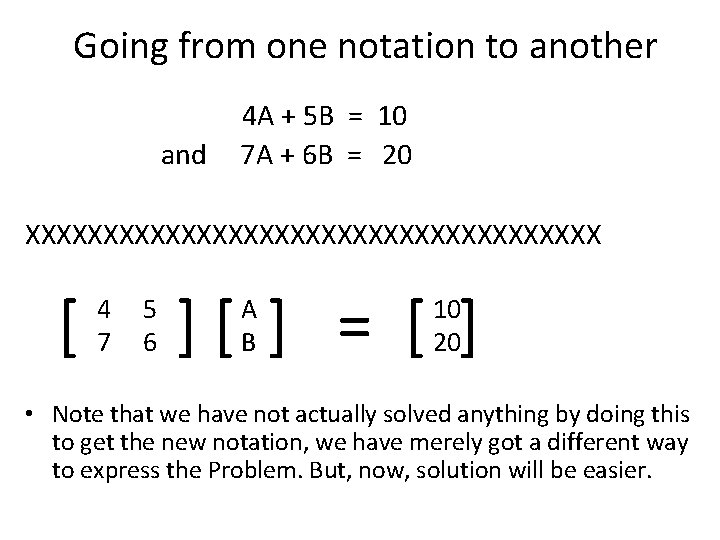Going from one notation to another and 4 A + 5 B = 10 7 A + 6 B = 20 XXXXXXXXXXXXXXXXXXX [ 4 7 5 6 ][ ] = [ ] A B 10 20 • Note that we have not actually solved anything by doing this to get the new notation, we have merely got a different way to express the Problem. But, now, solution will be easier.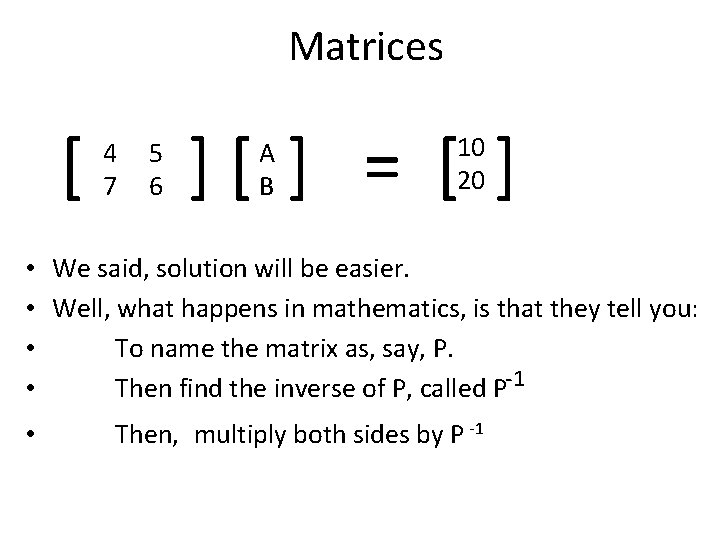Matrices [ 4 7 5 6 ][ ] = [ ] A B 10 20 • We said, solution will be easier. • Well, what happens in mathematics, is that they tell you: • To name the matrix as, say, P. • Then find the inverse of P, called P-1 • Then, multiply both sides by P -1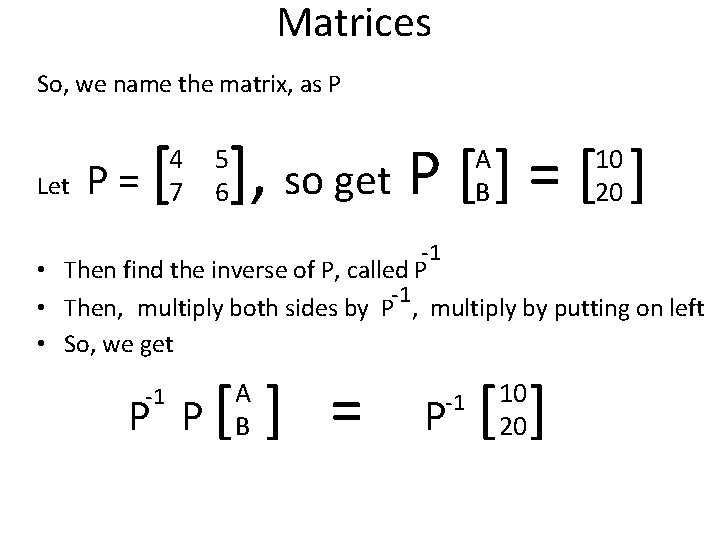Matrices So, we name the matrix, as P Let P= [ ], so get P [ ] = [ ] 4 7 5 6 A B 10 20 -1 • Then find the inverse of P, called P -1 • Then, multiply both sides by P , multiply by putting on left • So, we get P P[ -1 A B ] = -1 P [ ] 10 20Matrices • So, we have P P[ -1 A B ] = P -1 [ ] 10 20 Now, for some matrix facts: • 1) For any square matrix P, -1 P • 2) For any matrix or vector T, x P = Identity x T is = T • 3) For any square matrix P, if P is of dimension 2 x 2, then its inverse, P-1 , is also of dimension 2 x 2.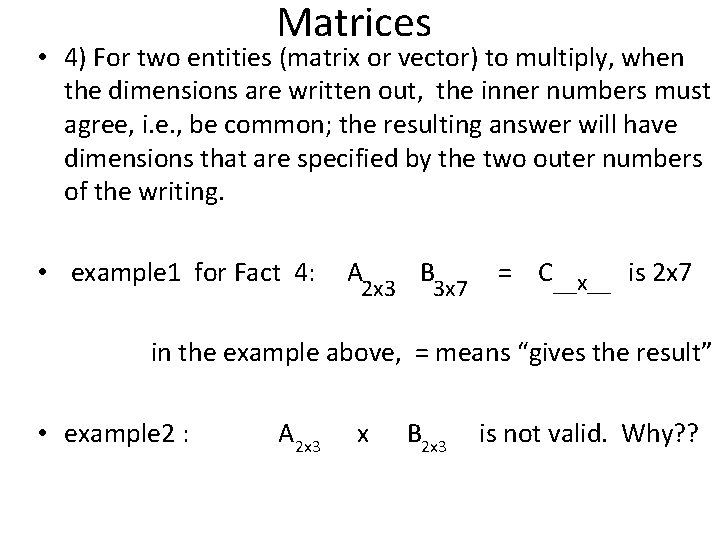Matrices • 4) For two entities (matrix or vector) to multiply, when the dimensions are written out, the inner numbers must agree, i. e. , be common; the resulting answer will have dimensions that are specified by the two outer numbers of the writing. • example 1 for Fact 4: A 2 x 3 B 3 x 7 = C__x__ is 2 x 7 in the example above, = means “gives the result” • example 2 : A 2 x 3 x B 2 x 3 is not valid. Why? ?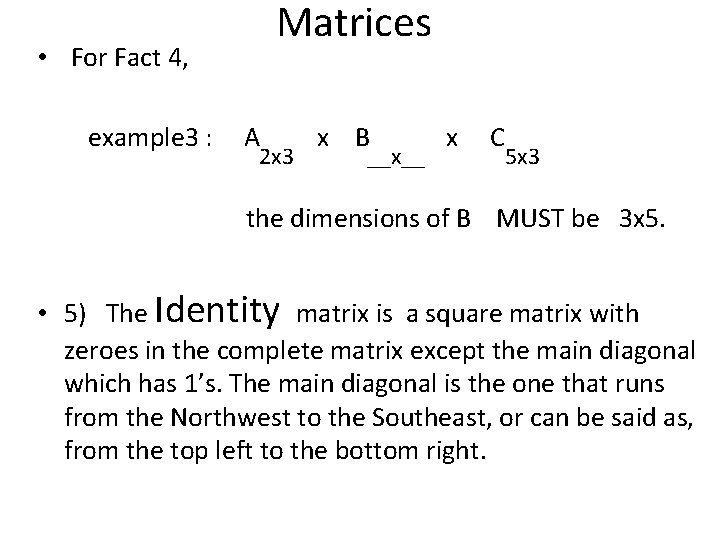Matrices • For Fact 4, example 3 : A 2 x 3 x B __x__ x C 5 x 3 the dimensions of B MUST be 3 x 5. • 5) The Identity matrix is a square matrix with zeroes in the complete matrix except the main diagonal which has 1’s. The main diagonal is the one that runs from the Northwest to the Southeast, or can be said as, from the top left to the bottom right.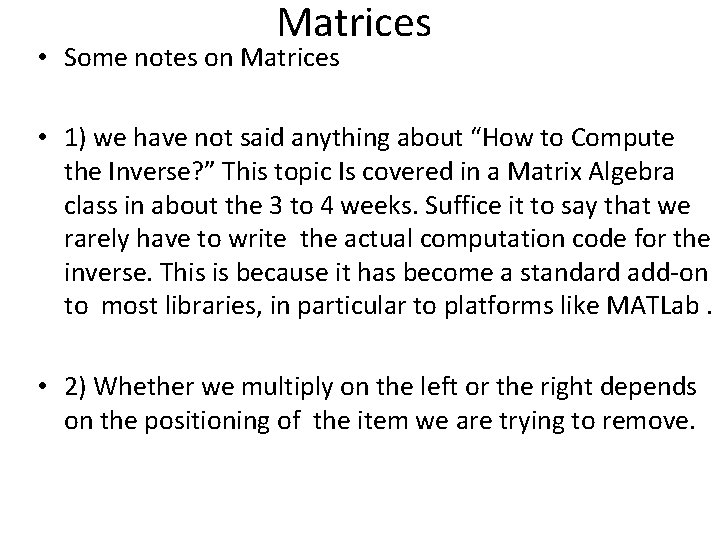Matrices • Some notes on Matrices • 1) we have not said anything about “How to Compute the Inverse? ” This topic Is covered in a Matrix Algebra class in about the 3 to 4 weeks. Suffice it to say that we rarely have to write the actual computation code for the inverse. This is because it has become a standard add-on to most libraries, in particular to platforms like MATLab. • 2) Whether we multiply on the left or the right depends on the positioning of the item we are trying to remove.